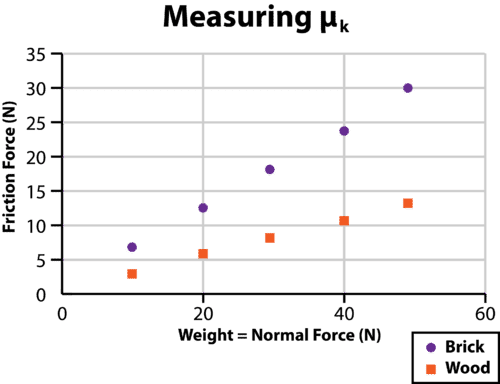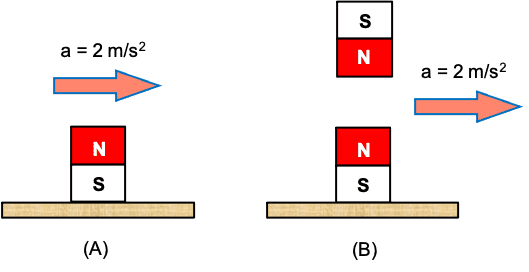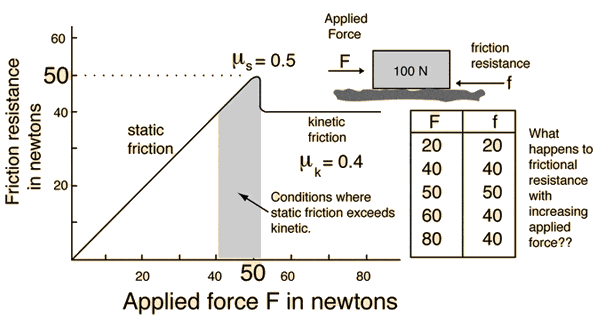# Coefficient of friction and normal force

• rudransh verma
furniture), the static friction (between the wardrobe and the floor) and the kinetic friction (between your hand and the wardrobe) are working together to move the wardrobe.f

#### rudransh verma

Gold Member
Homework Statement
If the normal reaction force is doubled, the coefficient of friction is-
Relevant Equations
##F_r(friction)=\mu F_N(normal)##
The answer should be no change but we know ##F=ma##. In this eqn when acceleration increases mass decreases for same force. So why not here? If normal is doubled ##\mu## should be halved.

The equation that you quoted is only an upper limit for the maximum force of static friction. The correct expression is ##f_{static}\leq \mu_s F_N##. Unlike its kinetic counterpart, the force of static friction does not have a specific value. It is whatever is necessary to provide the observed acceleration but up to a certain limit that it cannot exceed.

It's like going to a store to buy a $20.00 shirt with a credit card that has a$500.00 limit. You charge to the card whatever is necessary, $20.00, not$500.00 which is the maximum you can spend. If the credit card company increases the card's limit to $1,000.00, you will still pay$20.00 for the shirt. Increasing the limit of your credit does not increase the cost of the shirt. Likewise, increasing the normal force does not increase the static force that is necessary to provide the observed acceleration. It increases the upper limit that static friction can have.

On edit: This applies to static friction. For kinetic friction the equation is always valid. The coefficient of kinetic friction is a measure of how slippery the contact is. See post by @Lnewqban below for more.

Last edited:
•Lnewqban
Force is the cause, the acceleration of the mass is the effect.
Normally, the mass does not decreases when acceleration increases.

Note that the coefficient of friction is unit-less, being a coefficient of linear proportionality in a graph showing Friction force versus Normal force.The answer should be no change but we know F=ma. In this eqn when acceleration increases mass decreases for same force. So why not here? If normal is doubled μ should be halved.
I must warn you of something, my brethren, never say "mass" decreases or increases, not even in relativity (you might be having some inspiring notions that mass increases as the speed of an object reaches to the speed of light); people have become immensely intolerable to the change in mass.

•rudransh verma
I must warn you of something, my brethren, never say "mass" decreases or increases, not even in relativity (you might be having some inspiring notions that mass increases as the speed of an object reaches to the speed of light); people have become immensely intolerable to the change in mass.
I don’t understand what you said but I will laugh!

•Hall
increasing the normal force does not increase the static force that is necessary to provide the observed acceleration. It increases the upper limit that static friction can have.
What is the context ?
Because if you increase the weight of the body then it will require more force to accelerate the body because of the increase in upper limit of static friction as you said.

So why not here? If normal is doubled ##\mu## should be halved.
Why are you assuming the (kinetic) friction remains the same if the normal force doubles?

What is the context ?
Because if you increase the weight of the body then it will require more force to accelerate the body because of the increase in upper limit of static friction as you said.
The problem says nothing about increasing the mass (or weight) of a body. To clarify the idea in your mind, please answer the following two questions that I made just for you.

In figure (A) below left, you see a magnet that is placed on a wooden plank. A force (not shown) is pulling the plank to the right so that the block + plank system is accelerating to the right with acceleration 2 m/s2. The coefficient of static friction is μs = 0.60 and the mass of the magnet is 0.10 kg. Assume g = 10 m/s2.
Question 1. Find the normal force and the force of static friction exerted by the plank on the magnet.

In figure (B) below right, you have the same situation except that an identical magnet is accelerating (under its own power) with the same acceleration so that it always stays above the magnet on the plank. The top magnet exerts a repulsive magnetic force of 10 N on the bottom magnet.
Question 2. Find the normal force and the force of static friction exerted by the plank on the magnet.•Lnewqban
This diagram explains what you feel when you push a heavy piece of furniture on a tile floor by yourself.

The heavy wardrobe does not start sliding until you exert certain amount (sometimes a lot) of pushing force: that is static friction, combined with the third law of Newton (the piece of furnisher pushes you back only as hard as you push on it, no more no less).

Once the thing start moving (the “grip” has been overcome by your pushing force), and if you keep pushing on it, you feel a little less of resistance while it slides forward: that is kinetic friction, which value is less than the static friction.

http://hyperphysics.phy-astr.gsu.edu/hbase/frict2.htmlHomework Statement:: If the normal reaction force is doubled, the coefficient of friction is-
Relevant Equations:: ##F_r(friction)=\mu F_N(normal)##

The answer should be no change but we know ##F=ma##. In this eqn when acceleration increases mass decreases for same force. So why not here? If normal is doubled ##\mu## should be halved.
Where does it say that the friction force stays constant?

Rewriting the question with ##F=ma## in mind:

If the acceleration is doubled, the mass is-

The answer should be no change. Nowhere is it specified that ##F## cannot change.

•rudransh verma
Homework Statement:: If the normal reaction force is doubled, the coefficient of friction is-
Relevant Equations:: ##F_r(friction)=\mu F_N(normal)##

The answer should be no change but we know ##F=ma##. In this eqn when acceleration increases mass decreases for same force. So why not here? If normal is doubled ##\mu## should be halved.
The statement to be completed is: (emphasis added)

If the normal reaction force is doubled, the coefficient of friction is __________ .

This is not referring to friction itself, but refers to the coefficient of friction.

The answer should be no change. Nowhere is it specified that F cannot change.
You are right! For single body this thing doesn’t apply ,only for two or more bodies. I was confusing between one and two bodies.

Homework Statement:: If the normal reaction force is doubled, the coefficient of friction is-
Relevant Equations:: ##F_r(friction)=\mu F_N(normal)##

The answer should be no change but we know ##F=ma##. In this eqn when acceleration increases mass decreases for same force. So why not here? If normal is doubled ##\mu## should be halved.
If you have ##a=bc## and all you know is that ##c## is increased, then you don't know what happens to ##a## or ##b## without some additional information. If we know ##b## is constant, then we can infer that ##a## is proportional to ##c##. Thus if ##c## is doubled then ##a## is also doubled.

On the other hand, if ##a## is constant then we know ##b## is inversely proportional to ##c##. Thus if ##b## is doubled, ##c## is halved.

In the first example involving ##f=\mu N##, we know from experience that ##\mu## is constant.

In the second example involving ##F=ma## you told us that ##F## is constant.

••rudransh verma and Lnewqban
In the first example involving ##f=\mu N##, we know from experience that ##\mu## is constant.
In other words, ##\mu## is independent of ##F_N##. That is why when you look up tables of coefficients of friction between various materials, the normal force is not mentioned and can be anything.

•rudransh verma